### The Best Card Trick?

##### Age 11 to 16 Challenge Level:

Time for a little mathemagic! Choose any five cards from a pack and show four of them to your partner. How can they work out the fifth?### Divisibility Tests

##### Age 11 to 16### Tournament Scheduling

##### Age 11 to 16

Scheduling games is a little more challenging than one might desire. Here are some tournament formats that sport schedulers use.### The Random World

##### Age 11 to 18

Think that a coin toss is 50-50 heads or tails? Read on to appreciate the ever-changing and random nature of the world in which we live.### Ding Dong Bell

##### Age 11 to 18

The reader is invited to investigate changes (or permutations) in the ringing of church bells, illustrated by braid diagrams showing the order in which the bells are rung.##### Age 11 to 18

Infinity is not a number, and trying to treat it as one tends to be a pretty bad idea. At best you're likely to come away with a headache, at worse the firm belief that 1 = 0. This article discusses the different types of infinity.### Mouhefanggai

##### Age 14 to 16

Imagine two identical cylindrical pipes meeting at right angles and think about the shape of the space which belongs to both pipes. Early Chinese mathematicians call this shape the mouhefanggai.### The Chinese Remainder Theorem

##### Age 14 to 18

In this article we shall consider how to solve problems such as "Find all integers that leave a remainder of 1 when divided by 2, 3, and 5."### Proofs with Pictures

##### Age 14 to 18

Some diagrammatic 'proofs' of algebraic identities and inequalities.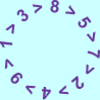### Whole Number Dynamics I

##### Age 14 to 18

The first of five articles concentrating on whole number dynamics, ideas of general dynamical systems are introduced and seen in concrete cases.### Some Circuits in Graph or Network Theory

##### Age 14 to 18

Eulerian and Hamiltonian circuits are defined with some simple examples and a couple of puzzles to illustrate Hamiltonian circuits.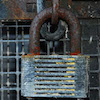### Keeping it Safe and Quiet

##### Age 14 to 18

Simon Singh describes PKC, its origins, and why the science of code making and breaking is such a secret occupation.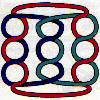##### Age 14 to 18

Some puzzles requiring no knowledge of knot theory, just a careful inspection of the patterns. A glimpse of the classification of knots, prime knots, crossing numbers and knot arithmetic.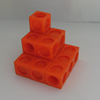### Sums of Powers - A Festive Story

##### Age 14 to 18

A story for students about adding powers of integers - with a festive twist.### The Use of Mathematics in Computer Games

##### Age 16 to 18

An account of how mathematics is used in computer games including geometry, vectors, transformations, 3D graphics, graph theory and simulations.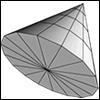### Conic Sections

##### Age 16 to 18

The interplay between the two and three dimensional Euclidean geometry of conic sections is explored in this article. Suitable for students from 16+, teachers and parents.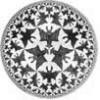### How Many Geometries Are There?

##### Age 16 to 18

An account of how axioms underpin geometry and how by changing one axiom we get an entirely different geometry.### Public Key Cryptography

##### Age 16 to 18

An introduction to the ideas of public key cryptography using small numbers to explain the process. In practice the numbers used are too large to factorise in a reasonable time.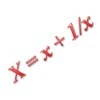### The Why and How of Substitution

##### Age 16 to 18

Step back and reflect! This article reviews techniques such as substitution and change of coordinates which enable us to exploit underlying structures to crack problems.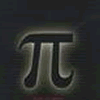### Approximations, Euclid's Algorithm & Continued Fractions

##### Age 16 to 18

This article sets some puzzles and describes how Euclid's algorithm and continued fractions are related.### What Are Complex Numbers?

##### Age 16 to 18

This article introduces complex numbers, brings together into one bigger 'picture' some closely related elementary ideas like vectors and the exponential and trigonometric functions and their derivatives and proves that e^(i pi)= -1.### Where Do We Get Our Feet Wet?

##### Age 16 to 18

Professor Korner has generously supported school mathematics for more than 30 years and has been a good friend to NRICH since it started.### Mathematics in the Financial Markets

##### Age 16 to 18

Financial markets mean the business of trading risk. The article describes in simple terms what is involved in this trading, the work people do and the figures for starting salaries.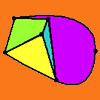### Euler's Formula

##### Age 16 to 18

Some simple ideas about graph theory with a discussion of a proof of Euler's formula relating the numbers of vertces, edges and faces of a graph.### Fractional Calculus I

##### Age 16 to 18

You can differentiate and integrate n times but what if n is not a whole number? This generalisation of calculus was introduced and discussed on askNRICH by some school students.### Infinite Continued Fractions

##### Age 16 to 18

In this article we are going to look at infinite continued fractions - continued fractions that do not terminate.